# How covariances behave: some intuitive views on normal distributions and Gaussian Processes¶

The goal of this post is to complement the classical material on Gaussian Processes and distributions, illustrating them with basic observations that speak to logical or geometric intuition.

I've found that most introductions to Gaussian Processes start from an abstract definition, then quickly dive into matrix computations or high-level math. Such topics are important and interesting, but may leave the newcomer without a tangible understanding of how Gaussian Processes behave. This post aims to address that need; that said, it does assume some basic mathematical background, especially in linear algebra and probability.

This post is not an introduction to Gaussian Processes, rather a complement to such introductions. For good introductions, I'd recommend to start with chapter 45 of MacKay's ITILA, then the first chapters of the GPML book by Rasmussen and Williams.

This post uses some SciPy libraries to display the examples (source code here):

In :
import gp_intuitive_views as gpv


## Cast of characters¶

• $\mathcal{X} = \{x \dots\}$: the index set of our Gaussian Process, which elements could be points in time, spatial coordinates, text strings... this set is usually infinite and continuous.
• $(T_x)_{x \in \mathcal{X}}$: our Gaussian Process, a family of real-valued random variables indexed by $\mathcal{X}$, any finite subset of which is Gaussian; viewed differently, a random variable in the space of functions from $\mathcal{X}$ to $\mathbb{R}$. In this article's examples, $T_x$ is always assumed to be a temperature (in Kelvins °K).
• $\mu: x \mapsto \mu(x) = \mathbb{E}[T_x]$, the mean function associated with $(T_x)_x$.
• $Z_x := (T_x - \mu(x))$, the difference from the mean. Like $T_x$ but with zero mean.
• $k: (x, x') \mapsto k(x, x') = \mathbb{E}[Z_x Z_{x'}]$, the covariance function or kernel function associated with $(T_x)_x$.
• $\Theta$: parameters of the functions $k$ and $\mu$. Often consists of characteristic lengthscales $l_i$ and output scales $T_V$.
• $\{x^{(1)}, \dots, x^{(n)}\} \subset \mathcal{X}$: a subset of the index points of particular interest, corresponding for example to our training dataset or to our test points.
• $\mathbf{K} := [k(x^{(i)}, x^{(j)})]_{ij}$, the covariance matrix obtained from applying kernel $k$ to points $\{x^{(1)}, \dots, x^{(n)}\}$.

## Introduction and perspectives¶

#### Definition¶

As you can read in many places, a Gaussian Process is a family $(T_x)_{x \in \mathcal{X}}$ of real-valued random variables, such that for any subset $\{x^{(1)}, \dots, x^{(n)}\}$ of $\mathcal{X}$, the random variables $T_{x^{(1)}}, \dots, T_{x^{(n)}}$ are jointly Gaussian, i.e the random vector $[T_{x^{(1)}}, \dots, T_{x^{(n)}}]$ follows a multivariate normal distribution.

Here $\mathcal{X}$ is called the index set of the GP, and can really be just any set (its element could be points in time, geographic coordinates, text strings, ...).

#### Illustration with physical quantities¶

In order to prevent confusion, it can be helpful to reason with physical dimensions. Throughout this article, all $T_x$ values are assumed to be temperatures (in Kelvins °K).

#### Probabilities of functions¶

A well-known and insightful viewpoint is that a Gaussian Process defines a probability distribution over the space of functions from $\mathcal{X}$ to $\mathbb{R}$. From that perspective, $T_x$ is not a random number; it's the evaluation of a random function at a given point $x \in \mathcal{X}$; you could write it $T(x)$ rather than $T_x$, and treat $T$ as the random variable.

Because the $(T_x)_x$ are jointly Gaussian, their means and covariances induce deterministic functions defined on $\mathcal{X}:$

$$\mu(x) := \mathbb{E}[T_x]$$$$k(x, x') := \text{cov}(T_x, T_{x'}) = \mathbb{E}[(T_x - \mu(x))(T_{x'} - \mu(x'))]$$

$\mu : \mathcal{X} \to \mathbb{R}$ is called the mean function, and $k : \mathcal{X}\times\mathcal{X} \to \mathbb{R}$ is called the covariance function or kernel. The function-space probability distribution is fully determined by these 2 functions.

To keep notation lightweight, it will be convenient to introduce the quantity:

$$Z_x := T_x - \mu(x)$$

which is the 'difference from expected temperature'. Observe that the $(Z_x)_{x \in \mathcal{X}}$ are also jointly Gaussian, with the same covariances as the $(T_x)_{x \in \mathcal{X}}$, but mean zero. Whereas the $(T_x)_x$ are absolute temperatures, the $(Z_x)_{x}$ are relative ones.

In Machine Learning applications, we usually start from the kernel and mean functions: we choose mean and kernel functions $\mu$ and $k$, thus choosing a probabilistic model, and then use it to do inference from observations. What's more, $\mu$ and $k$ are usually defined with parameters, and part of the work consists of finding 'optimal' values of these parameters, or a posterior distribution for them, given training data. See Appendix B for concrete details.

It bears repeating that the 'index set' $\mathcal{X}$ can be any set. In particular, any multivariate normal distribution over $\mathbb{R^n}$ is a Gaussian Process, one with a finite discrete index set of size $n$.

We're often accustomed to writing vectors and matrices with integer indexes, thinking of them as numerical arrays. I would like you to open your mind a bit, and think of vectors and matrices as real-valued functions of their indices. So the vector $[T_{x^{(1)}}, \dots, T_{x^{(n)}}]$ is really a function $x^{(i)} \mapsto T_{x^{(i)}}$, defined over the finite domain $\{x^{(1)}, ... x^{(n)}\}$.

One final piece of advice: know your spaces! Our discussion will involve operations / navigation in various spaces:

• the index set $\mathcal{X}$, and its representations
• the 'values' space $\mathbb{R}$,
• the infinite-dimensional affine space of functions $x \mapsto T_x$,
• the infinite-dimensional vector space of functions $x \mapsto Z_x$,
• the finite-dimensional projections of the above 2 obtained by considering a finite subset of datapoints ${x^{(1)}, \dots, x^{(n)}}$
• the space of kernel parameters

When we talk about linear transformations / geometric representations / etc. it's important to keep track of what spaces we are operating in.

## Extreme covariances¶

### Zero covariance: no uncertainty¶

Let's start with an extreme case: when the covariance matrix is null, i.e when the covariance between any two of the $T_x$ is zero.

In this case, each of the $T_x$ is a (degenerate) Gaussian distribution with mean $\mu(x)$ an variance 0, a.k.a a 'constant' or 'deterministic' random variable, a.k.a a Dirac distribution $\delta_{\mu(x)}$.

In other words, if the covariance is always zero, there is no uncertainty: each of the $T_x$ is guaranteed to be equal to $\mu(x)$.

### Constant covariance: all $Z_x$ are the same¶

Let's now consider a slightly less degenerate case: when the covariance is constant, i.e there is a constant temperature $T_V$ such that for all $x, x'$:

$$\mathbf{K}_{x x'} = T_V^2$$

In other words, for any indices $x$ and $x'$, the covariance matrix of $[T_x, T_{x'}]$ is

$$\begin{bmatrix} T_V^2 & T_V^2 \\ T_V^2 & T_V^2 \end{bmatrix} = T_V^2 . \begin{bmatrix} 1 & 1 \\ 1 & 1 \end{bmatrix}$$

We can see that this matrix has a rank of 1, and that its column space is the direction generated by the single vector $\begin{bmatrix} 1 \\ 1 \end{bmatrix}$; this is precisely the space described by the equation $Z_x = Z_{x'}$. Let's plot a sample from this distribution with $T_V = 10°K$ to illustrate that:

In :
gpv.plot_constant_covariance_sample()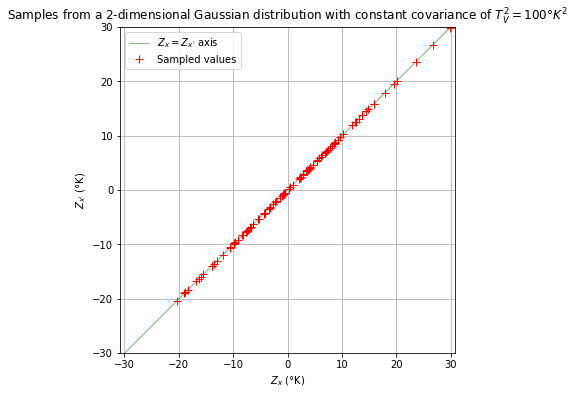It follows that if the covariance matrix of $[T_{x_1}, \dots, T_{x_n}]$ is constant, the $Z_{x_i} := (T_{x_i} - \mu(x_i))$ are constrained to all be equal. In other words, the difference from the mean $Z_x := (T_x - \mu(x))$ is uncertain, but constrained to be the same for all $x$. Effectively, the $Z_x$ are all the same random variable, a 0-mean Gaussian with standard deviation $T_V$. Note that this is a much stronger statement than "all $Z_x$ follow the same law"!

This special case suggests why, in continuous $\mathcal{X}$ index sets, continuous kernels yield continuous functions: as $x$ gets close to $x'$, the covariance matrix of $[T_x, T_{x'}]$ gets close to a constant matrix, and $Z_x \approx Z_{x'}$ with high probability.

### Rank-1 covariance: one $T_x$ to know them all¶

We have just seen that if the covariance matrix is constant, then it has rank 1, and all $Z_x$ are constrained to have the same value, i.e the random function $(x \mapsto Z_x)$ is constrained to be proportional to the constant function $(x \mapsto 1)$.

Generalizing a bit, given a prescribed function $(x \mapsto f(x))$, you might wonder the following: _can one design a covariance matrix such that the random function $(x \mapsto Z_x)$ is constrained to be proportional to $(x \mapsto f(x))$?_

It turns out that you can: denoting $\mathbf{f}$ the vector $[f(x^{(1)}), \dots, f(x^{(n)})]$, this is accomplished by a covariance matrix of the form

$$\mathbf{K} := T_V^2 . \mathbf{f} \mathbf{f}^\top$$

... in which $T_V$ is a charasteristic standard deviation constant. In other words, looking at a specific cell of this matrix:

$$\text{cov}(T_x, T_{x'}) = T_V^2 f(x) f(x')$$

The existence of such a decomposition is exactly equivalent to saying that $\mathbf{K}$ has rank 1, and that the column space of $\mathbf{K}$ is generated by $\mathbf{f}$. What's more, if $\mathbf{f}$ is normalized to have euclidean norm 1, then $T_V^2$ is the eigenvalue associated with vector $\mathbf{f}$.

Another way to see it is the following: effectively, even though $[T_{x^{(1)}}, \dots, T_{x^{(n)}}]$ is a multivariate Gaussian distribution, it has no more uncertainty than just one scalar Gaussian: all of the $T_x$ can be determined with certainty by knowing just one of them (not necessarily any one of them; consider what happens when $f(x) = 0$).

### Fixed-rank covariance: linear regression model¶

Generalizing again, let's now assume that the covariance matrix $\mathbf{K}$ has rank $d$.

In this case, the vector $[Z_{x^{(1)}}, \dots, Z_{x^{(n)}}]$ is constrained to live in a $d$-dimensional space, which is the column space of $\mathbf{K}$. As a result, all of the $T_x$ can be determined with certainty by knowing just $d$ of them (not any $d$ of them however - can you tell which $d$-sized subsets of the indices work?).

Because $\mathbf{K}$ is symmetric, its column space has an orthonormal basis of $d$ eigenvectors $\mathbf{f}^{(1)}, ..., \mathbf{f}^{(d)}$, from which $\mathbf{K}$ can be decomposed as follows:

$$\mathbf{K} = \sum_{i = 1}^{d} T_i^2 . \mathbf{f}^{(i)}\mathbf{f}^{(i) \top}$$

We can then say that the vector $[Z_{x^{(1)}}, \dots, Z_{x^{(n)}}]$ is constrained to be a linear combination of the $\mathbf{f}^{(i)}$; the coefficients of this linear combination are independent Gaussians, with variances given by the eigenvalues $T_i^2$.

Viewing these vectors as functions of their indices $x^{(i)}$, we can reformulate by saying that $(x \mapsto Z_x)$ is constrained to be a linear combination of the basis functions $(x \mapsto \mathbf{f}^{(i)}_x)$. This is equivalent to a linear regression model with $d$ basis functions.

### Diagonal and sparse covariance: independent variables¶

Gaussian distributions have the property that two jointly Gaussian variables $T_x$ and $T_{x'}$ are independent if and only if $\text{cov}(T_x, T_{x'}) = 0$.

As a consequence, the independence of a list of jointly Gaussian variables corresponds to their covariance matrix being diagonal.

For Gaussian Processes inference, a kernel that yields only diagonal covariance matrices would not be very useful, because training points would tell you nothing about test points. Having said that, noise is usually modeled by a diagonal matrix being added to the covariance matrix of the training data, the assumption being that noise consists of small independent Gaussian perturbations at all data points.

## Scale parameters, and stretching spaces¶

In this section, we'll look at kernels defined in terms of 'characteristic lengthscales', and describe how these lengthscales influence the probabilities of sampled functions.

As a illustration, we'll consider a kernel defined on a 1-dimensional index space $\mathcal{X} \cong \mathbb{R}$ (which would be relevant for modeling time series, for example), using a popular 'Squared-Exponential' kernel:

$$k_{(T_V, l)}(x, x') := T_V^2 \exp \left(- \frac{1}{2} \left(\frac{x - x'}{l}\right)^2 \right)$$

in which $T_V$ is an 'output scale' parameter, and $l$ is a 'lengthscale' parameter.

WARNING: do not interpret the above expression as a Gaussian probability density - it isn't one. We'll come back to this later.

Note the dimensions of parameters:

1. $T_V^2 = k(x, x) = \text{cov}(T_x, T_x) = \mathbb{E}[(T_x - \mu(x))^2] = \mathbb{E}[Z_x^2]$, and so the outer scale $T_V$ has the dimension of a temperature, justifying the notation.
2. $l$ has the dimension of a distance in the input space $\mathcal{X}$.

### Output scale: characteristic amplitude¶

Let's plot some samples of the above Gaussian GP, with various values of the output scale $T_V$:

In :
gpv.plot_outputscales_examples()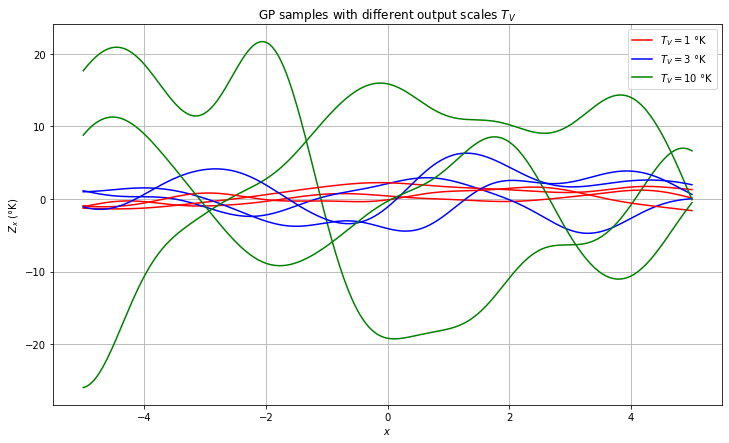As can be expected, the outputscale $T_V$ is proportional to the 'typical amplitude' of $Z_x$.

This can be understood by the following probabilistic argument. Recall that each kernel $k$ defines a probability distribution $\mathbb{P}_k$ on the space of functions $\mathbb{R}^\mathcal{X}$. Now, let $k_1, k_\alpha$ two kernel with outputscales respectively equal to $T_V$ and $\alpha T_V$. It can then be seen that the 'scaling' transformation of functions $(x \mapsto Z_x) \mapsto (x \mapsto \alpha Z_x)$, from $(\mathbb{R}^\mathcal{X}, \mathbb{P}_{k_0})$ to $(\mathbb{R}^\mathcal{X}, \mathbb{P}_{k_\alpha})$, is a transformation that preserves probability.

Essentially, if $Z$ is a typical function by kernel $k$, then $\alpha.Z$ is a typical function by kernel $\alpha.k$ (we don't define 'typical' here, but it's generally used to mean 'living in a subset of high probability').

### $\mathcal{X}$-space lengthscales¶

Let's now examine what happens when we vary the lengthscale $l$:

In :
gpv.plot_lengthscales_examples()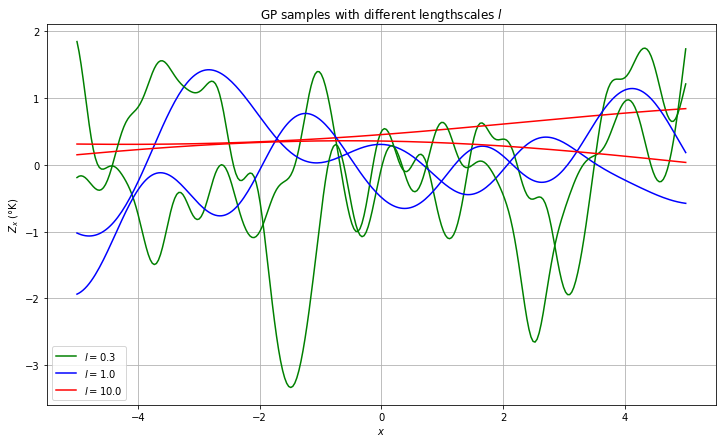We see here that $l$ yields a typical 'distance of variability' in $\mathcal{X}$-space. For instance, it can be proved that $l$ is proportional to the expected distance between 0-crossings of a sampled function.

A probabilistic argument similar to the above can be made: if $f: [x_0, x_1] \to \mathbb{R}$ is a 'typical' function for a kernel with lengthscale $l$, then $f_\alpha : [\alpha x_0, \alpha x_1] \to \mathbb{R}$ defined by $f_\alpha(x) := f(\alpha x)$ is a typical function for the same kernel with lengthscale modified to $\alpha l$.

## RBF kernels: "independence is distance"¶

In Gaussian Processes regression, choosing a covariance function means taking a prior on functions. Common assumptions are :

1. $T_x \approx T_{x'}$ if $x$ is "close" to $x'$: for instance, you don't expect temperature to vary by 20°C in one hour.
2. $T_x$ is independent from $T_{x'}$ if $x$ is "far" from $x'$; you don't expect the temperature in Paris right now to tell you much about the temperature in Hanoi two months later.
3. The individual variances at each point are roughly of the same magnitude.

With that in mind, covariance functions are often chosen to be of the form:

$$k(x, x') := T_V^2 f(d_{\Theta}(x, x'))$$

... in which:

• $(x, x') \mapsto d_{\Theta}(x, x')$ is a "distance" / "dissimilarity" function with parameters $\Theta$ (the parameters usually involve characteristic lengthscales),
• $r \mapsto f(r)$ is a positive function which is highest around $r = 0$ with $f(0) \approx 1$, and $\lim_{r \to +\infty} f(r) = 0$.
• $T_V$ is a standard deviation constant.

#### Example: the Squared Exponential kernel¶

Because of the expression for the Gaussian density, Gaussian Processes aficionados have developed a fondness for squared exponentials. As a consequence, a very popular choice for kernels of the above form is $f(r) = e^{- \frac{1}{2} r^2}$, which yields:

$$k(x, x') := T_V^2 e^{- \frac{1}{2} d_{\Theta}(x, x')^2}$$

CONFUSION PITFALL: although it contains something that looks very much like a Gaussian density, the above expression is not a probability density. For practical purposes, you should interpret the resemblance as a pure coincidence.

### Euclidean distance, lengthscales, and feature relevance¶

We now make a very common assumption about the index set $\mathcal{X}$: that each $x \in \mathcal{X}$ can be represented as a numerical vector of "features"

$$x = [x_1, \dots, x_D]$$

We then choose our $d_{\Theta}(x, x')$ distance to be a Euclidean norm, of the form:

$$d_{\Theta}(x, x')^2 := \sum_{i=1}^{D}{\left( \frac{x_i - x'_i}{l_i} \right)^2}$$

in which $\Theta = (l_i)_{i=1}^{D}$ are characteristic lengthscales associated with each feature. Our goal here is to study how the choice of characteristic lengthscales influences covariance, thus bridging geometric intuition to probabilities and inference.

### Huge lengthscale $\implies$ irrelevant feature¶

If $l_i$ is very high, i.e $l_i \gg |x_i - x'_i|$ for any $x, x'$ you may encounter, then $\left(\frac{x_i - x'_i}{l_i} \right) \approx 0$, and the $i$-th feature plays no role in computing the covariance. A very high lengthscale value makes the corresponding feature irrelevant.

During Gaussian Process training, when using an optimization algorithm to adjust the values of the kernel parameters, it's common to see some lengthscales go to very high values: it means that the algorithm is learning from the data that this feature is irrelevant.

### Tiny lengthscale $\implies$ independent layers¶

On the other hand, if $l_i$ is very small, i.e $l_i \ll |x_i - x'_i|$ whenever $x_i \neq x'_i$, then the $i$-th drives the covariance between $Z_x$ and $Z_{x'}$ to zero: a tiny lengthscale makes the corresponding feature split the data into independent layers.

In particular, if all lengthscales become very small, then the covariance matrix of the points you consider becomes diagonal, and all points become independent.

### Lengthscales, features mapping, and geometry¶

Another way to look at lengthscales is to view them as acting on the features rather than on the distance: a given choice of lengthscales $(l_i)_{i=1}^D$ transforms each feature vector $x = [x_1, \dots, x_D]$ into a dimension-less vector $u := [\frac{x_1}{l_1}, \dots, \frac{x_1}{l_D}]$ of $\mathbb{R}^D$; the distance that is applied to these vectors is then the usual isotropic Euclidean distance:

$$d(u, u')^2 = \sum_{i=1}^D{(u_i - u'_i)^2}$$

Changing the lengthscales then changes how the $u$ data points are 'stretched' in $\mathbb{R}^D$: decreasing $l_i$ strechtes the point further apart along the corresponding dimension, whereas driving $l_i$ to infinity 'collapses' the points onto the $u_i = 0$ hyperplane. Let's visualize an example with 2 features:

In :
gpv.demo_lengthscales_in_2d_u_space()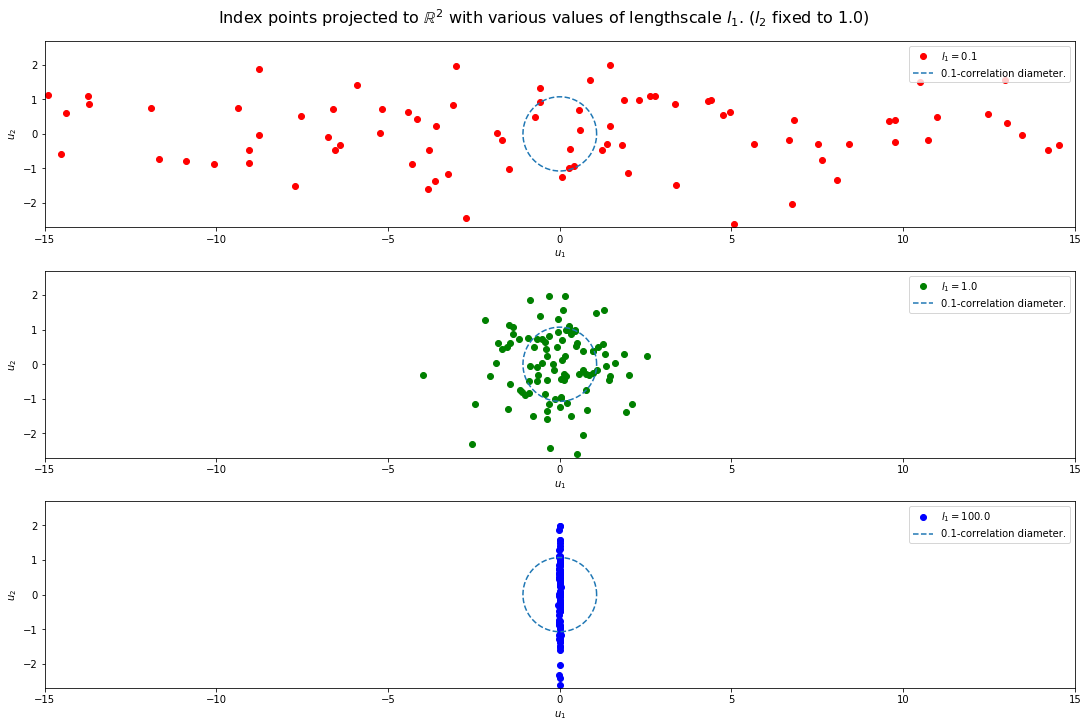In the above figure, the drawn circle has a diamater corresponding to a correlation of 0.1 by the SE kernel.

### Example: periodic kernel with decay¶

Here we illustrate on an example how geometrical thinking can help understand kernels.

Imagine we're modeling a time series $(T_t)_t$ that exhibits a short-term near-periodic trend, and a long-term non-periodic trend. For instance, the month-averaged temperature in Paris has a 1 year periodic trend on the short term (because seasons), and a long-term non-periodic trend (because global warming) :We can model this as a Gaussian Process using the following kernel:

$$k(t, t') = T_V^2 \exp{\left(-\frac{1}{2}\left[\left(\frac{1}{\pi }\frac{t_Y}{t_P}\sin\left(\pi \frac{t - t'}{t_Y}\right)\right)^2 + \left(\frac{t - t'}{t_L}\right)^2 \right]\right)}$$

in which $t_Y = \text{1 year}$, $t_L$ is a characteristic timescale corresponding to the long-term trend, and $t_P$ is a characteristic timescale corresponding to the periodic trend. The parameters that you'd want to adjust are $t_P, t_L$ and $T_V$. Reasonable settings would be $t_P \ll t_Y \lt t_L$.

Inside the brackets, the first term $\left(\frac{1}{\pi }\frac{t_Y}{t_P}\sin\left(\pi \frac{t - t'}{t_Y}\right)\right)^2$ corresponds to a 'periodic' time gap (by which $t$ and $t'$ are 'close' if they are approximately the same time of the year), whereas the second term $\left(\frac{t - t'}{t_L}\right)^2$ corresponds to an ordinary time gap.

The periodic term can be understood as follows: project each time $t$ to the unit circle at $c(t) := \left[\cos\left(2 \pi \frac{t}{t_Y}\right), \sin\left(2 \pi \frac{t}{t_Y}\right)\right]$, then compute the euclidean squared distance $\frac{1}{\theta_P^2}\lVert c(t) - c(t') \rVert^2$, in which $\theta_P$ is a characteristic 'angular' scale to be compared to the 'full revolution' $2 \pi$; because timescales are more intuitive than angular scales, we then introduce $t_P := \frac{\theta_P}{2\pi}t_Y$, and after some trigonometric manipulations we obtain the formula given above. So in effect, when computing the 'periodic distance', we're just computing ordinary euclidean distance, but after having projected the timeline to the unit circle.

Just as the periodic term corresponds to projecting the timeline onto a circle, it can be seen the entire 'distance' expression inside the brackets corresponds to projecting the timeline onto a spiral, that 'wraps around' a cylinder in 3D space:

In :
gpv.plot_spirals()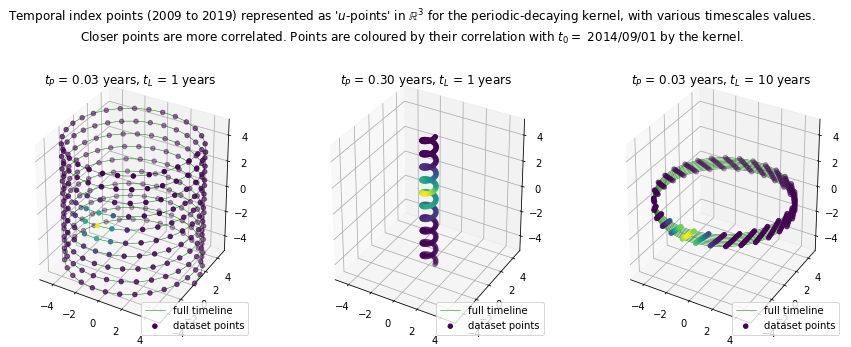### Lengthscales, spheres of influence, and model complexity¶

Note: I would appreciate suggestions from experts to better formalize the ideas of this section.

The above examples suggest the idea that each index point $x \in \mathcal{X}$ has a kind of 'sphere of influence' - a neighbouring region of points with significant covariance to $x$.

As model parameters such as lengthscales vary, so does the volume emcompassed by such spheres of influence. If this volume becomes very small, all points of the dataset are modeled as independent. If it becomes very large, one sphere of influence is sufficient to cover all your dataset, and the temperature at one point determine it at all the others.

You might then ask: "how many of these spheres of influence does it take to cover my dataset?". Vaguely speaking, the number of 'independent regions' in which you can partition your training points, which you might interpret as the number of 'degrees of freedom' of your prior distribution, or the number of 'arbitrary decisions' which resulted in your training data, or the 'entropy' of your distribution - all things you might relate to model complexity.

This is something we can relate to the Bayesian notion of evidence. In the case of Gaussian Processes, assuming our observed data consists of the vector $\mathbf{Z} = [Z_{x^{(1)}}, \dots, Z_{x^{(n)}}]$, the evidence for parameters value $\Theta$ is:

$$\log(\text{ev}(\Theta)) := \log{\mathbb{P}(\mathbf{Z} | \Theta)} = -\frac{1}{2}\mathbf{Z}^\top\mathbf{K}_\Theta^{-1}\mathbf{Z} -\frac{1}{2}\log{|2\pi . \mathbf{K}_\Theta|}$$

in which $\mathbf{K}_\Theta$ is the covariance matrix yielded by the kernel $k_\Theta$ on the observed points $(x^{(i)})_{i=1}^n$.

The first term can be interpreted as a data-fit term, and the second term can be interpreted as a complexity penalty term. The principle of Occam's Razor tells us that we should strike a balance between data-fit and model complexity, and for a given data-fit prefer models of low complexity.

## Composition of kernels¶

### Kernel Products: correlation by logical AND¶

One interesting feature of kernels is that it's possible to compose them by various operations. One of them is the product:, given 2 kernels $k_1$, $k_2$, you can make the 'product' kernel:

$$k_\Pi(x, x') := k_1(x, x') k_2(x, x')$$

Notice the following property: $k_\Pi(x, x') \approx 0$ (i.e $T_x$ and $T_{x'}$ are significantly independent according to $k_\Pi$), if and only if either $k_1(x, x') \approx 0$ or $k_2(x, x') \approx 0$. In other words, a product kernel models correlation as the logical AND of correlation by each factor.

Notice also that, thanks to the algebraic properties of the exponential function and Euclidean norm, the Squared-Exponential kernel can be viewed as a product of kernels:

$$k_{(l_i)_i}^{\text{SE}}(x, x') := \exp\left(-\frac{1}{2}\sum_{i=1}^{D}{\left( \frac{x_i - x'_i}{l_i} \right)^2}\right) = \prod_{i=1}^{D}{\exp\left(-\frac{1}{2}\left( \frac{x_i - x'_i}{l_i} \right)^2\right)} = \prod_{i=1}^{D}{k_{l_i}^{\text{SE}}(x_i, x'_i)}$$

In our periodic-decaying example, this can be read as: _"$T_t$ and $T_{t'}$ are correlated when $t$ and $t'$ are approximately at the same time of the year AND their respective years are not too far apart."_

So we see that when using an RBF kernel, we can compose features via the distance function or via a product of feature-specific kernels. It just so happens that with the Squared-Exponential kernel both strategies are equivalent, but with other kernels you will have to make a choice.

### Kernel Sums: correlation by logical OR¶

Just like we can multiply kernels, we can also sum them:

$$k_{\Sigma}(x, x') := k_1(x, x') + k_2(x, x')$$

Making the common assumption that $k_1$ and $k_2$ don't take significant negative values, a similar property to the above follows: a sum kernel models correlation as the logical OR of correlation by each term.

## Appendix A: normal distributions¶

This section is a refresher about multivariate normal distributions. We start with a restricted definition, that we will generalize later:

### Multivariate normal distribution: non-degenerate definition¶

A non-degenerate multivariate normal distribution is a probability distribution over $\mathbb{R}^n$ that has a probability density of the form:

$$\mathbb{P}(x) = K_{S} \exp\left(-\frac{1}{2}(x - \mu)^\top S^{-1} (x - \mu) \right)$$

in which:

• $\mu \in \mathbb{R}^n$ is a vector (the mean of the distribution)
• $S \in \mathcal{M}_{n}(\mathbb{R})$ is a positive definite symmetric matrix, the covariance matrix of the distribution.
• $K_S$ is a normalization constant of no interest for this article.

Such a distribution has the property that $\mathbb{E}[x] = \mu$ and $\mathbb{E}[xx^\top] = S$, which is why these quantities are called mean and covariance.

This definition is restrictive, in the sense that we require that the matrix $S$ be invertible, i.e definite. It's interesting to generalize this definition to the semidefinite-positive case, as it will provide insightful behaviours at the limit of the degenerate case.

To do that, we need a bit of linear algebra background. A symmetric matrix, being diagonizable, stabilizes its column space (a.k.a the 'image' of the matrix), and restricted to its column space, is invertible (for $b \in \text{colsp}(S)$, the equation $Sx = b$ has a unique solution $x \in \text{colsp}(S)$).

Then we can define a normal distribution from $S$ by saying that all of the probability mass is on its column space (1), and that restricted to its column space, the probability density is of the above form. This leads us to:

### Multivariate normal distribution: general definition¶

A multivariate normal distribution is a probability distribution $\mathbb{P}$ over $\mathbb{R}^n$, such that:

• there exists an affine subspace $\mathcal{E} \subset \mathbb{R}^n$ concentrating all the probability mass: $\mathbb{P}[x \notin \mathcal{E}] = 0$,
• the restriction of $\mathbb{P}$ to $\mathcal{E}$ is a non-degenerate normal distribution.

So for example, a degenerate 3D normal distribution could concentrate all of the probability mass on a 2D plane, or a 1D line, or even on a single point (in this last case there is no uncertainty).

Note that the mean and covariance matrix are still defined for a degenerate normal distribution, by $\mu = \mathbb{E}[x]$ and $S = \mathbb{E}[xx^\top]$. It's just that we can no longer write a probability density $\mathbb{P}(x)$ based on them.

(1) Strictly speaking, the probability mass is on $\mu + \text{colsp}(S)$, which is an affine subspace of $\mathbb{R}^n$.

## Appendix B: Gaussian Processes regression¶

Assume that we want to predict temperature at the test points $\{x_*^{(1)}, \dots, x_*^{(m)}\}$, and that we have observed noisy temperatures $\{Y_1, \dots, Y_n\}$ at the training points $\{x^{(1)}, \dots, x^{(n)}\}$, where the measurements are made with Gaussian noise of standard deviation $\sigma$.

Then we can infer that given the observed data, the test vector $\mathbf{T_*} := [T_{x_*^{(1)}}, \dots, T_{x_*^{(m)}}]$ follows a multivariate normal distribution, with mean and covariance given by:

$$\mathbb{E}[\mathbf{T_*}] = \begin{bmatrix} \mu(x_*^{(1)}) \\ \vdots \\ \mu(x_*^{(m)}) \end{bmatrix} + \begin{bmatrix} k(x_*^{(1)}, x^{(1)}) & \dots & k(x_*^{(1)}, x^{(n)}) \\ \vdots & \ddots & \vdots \\ k(x_*^{(m)}, x^{(1)}) & \dots & k(x_*^{(m)}, x^{(n)}) \end{bmatrix} \left( \begin{bmatrix} k(x^{(1)}, x^{(1)}) & \dots & k(x^{(1)}, x^{(n)}) \\ \vdots & \ddots & \vdots \\ k(x^{(n)}, x^{(1)}) & \dots & k(x^{(n)}, x^{(n)}) \end{bmatrix} + \sigma^2 . \mathbf{I}_n \right)^{-1} \left(\begin{bmatrix} Y_1 \\ \vdots \\ Y_n \end{bmatrix} - \begin{bmatrix} \mu(x^{(1)}) \\ \vdots \\ \mu(x^{(n)}) \end{bmatrix} \right)$$$$= \mathbf{\mu_*} + \mathbf{k_*}^\top(\mathbf{K} + \sigma^2 . \mathbf{I}_n)^{-1}(\mathbf{Y} - \mathbf{\mu})$$$$\text{cov}(\mathbf{T_*}) = \begin{bmatrix} k(x_*^{(1)}, x_*^{(1)}) & \dots & k(x_*^{(1)}, x_*^{(m)}) \\ \vdots & \ddots & \vdots \\ k(x_*^{(m)}, x_*^{(m)}) & \dots & k(x_*^{(m)}, x_*^{(m)}) \end{bmatrix} - \mathbf{k_*}^\top(\mathbf{K} + \sigma^2 . \mathbf{I}_n)^{-1}\mathbf{k_*}$$